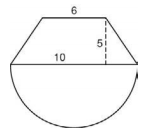# Area of Composite Figures

In earlier grades, you learned how to find the area of rectangles, triangles, and parallelograms. In this lesson, we will use our knowledge of finding area to find the area of figures composed of multiple shapes. These composed figures are often called irregular figures or composite figures. In most cases, you can separate the composite figures into simpler shapes whose areas you can find, and then add these areas together. It is important to note that these simple shapes may not overlap.

Below are some of the area formulas that you will be using.

SHAPE AREA FORMULA
Circle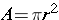Parallelogram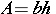RectangleSquareTrapezoidTriangle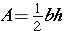Many times, there will be more than one way to separate the figures into simpler shapes.You can draw two vertical lines to break apart this shape into 3 different rectangles.

The rectangle on the left is 50 cm by 20 cm. What is its area?

The middle rectangle has dimensions of 40 cm by 30 cm. Do you see how we got those measures? (50 – 20)

Finally, the rectangle at the right is going to be 10 by 20. We can see that we have used 20 and 40 out of the total 70 cm for the width – that leaves 10.

The areas of the three rectangles are: 1000, 1200, and 200.

When we add these areas, we get 2400 sq cm.

Let us break apart the figure above into three rectangles by drawing two horizontal lines.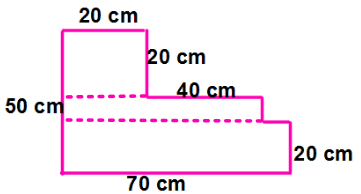The dimensions of the top rectangle would be 20 by 20.

The middle rectangle would have dimensions of 60 by 10.

The bottom rectangle would measure 70 by 20 cm.

The areas of the three rectangles are: 400, 600, and 1400.

When we add these areas, we get 2400 sq cm.

In an earlier course, you learned to find the area of a shape on the coordinate plane. To review, when an irregular figure is drawn on graph paper, you can estimate its area by counting whole squares and parts of squares.

Here is the strategy:

1. Count the number of whole squares.
2. Combine parts of squares to make whole squares orsquares.
3. Add the whole and partial squares and estimate the area.

*Multiply the number of whole or almost whole squares by 1 and multiply the number of half or almost-half squares by 0.5.What is the area of the figure above? (Did you get about 22 square units?) Great!

Take a look at the video to see more examples for finding area of composite figures.

## Area of Composite Figures Practice

#### Find the area for each figure.

1.2.